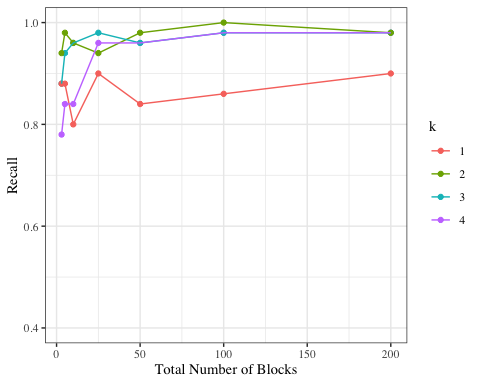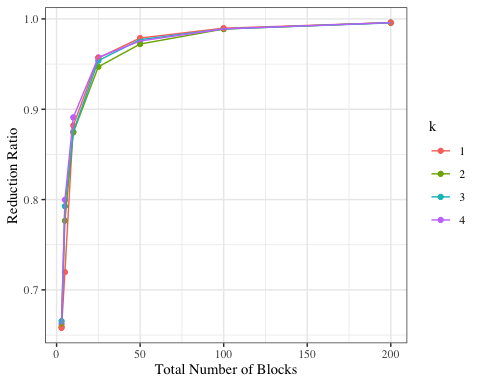# klsh

#### 2020-10-14

We present a small example from Steorts, R., Ventura, S., Sadinle, M., and Fienberg, S. (2014). “Blocking Comparisons for Record Linkage.” Privacy in Statistical Databases (Lecture Notes in Computer Science 8744), ed. J Domingo-Ferrer, Springer, 252-268, . We will be using the blink package in R and the RLdata500 data set, which was previously available in the Record Linkage package (but has been deprecated).

In a record linkage task one wants to remove duplicate entries from multiple databases. However, before performing this task, one needs to perform a means of dimension reduction so that the record linkage task is computationally scalable.

Using the KLSH algorithm, we illustrate an example of using this package using a German dataset comprised of first and last name and full date of birth.

Our goals include

• Presenting the RLdata500 dataset with summary information.
• Illustrating how we can format the RLdata500 dataset to work with the klsh
• Running KLSH on the RLdata500 data set to create blocks
• Explaining the tunning parameters of KLSH and how to choose these in practice with evaluation metrics.
• Sample output and visualizations

## Understanding the RLdata500 dataset

The RLdata500 dataset exists already in the blink package in R. We review this data set for the user.

The RLdata500 data consists of 500 records with 10 percent duplication. Thus, there are 450 unique individuals. There is full information on each record containing first name, last name, and full date of birth.

We first load the blink package and load the RLdata500 data set. We also, provide the first few lines of the data. We also remove missing values (they are all missing in this data set).

library(blink)
library(plyr)
library(klsh)
data(RLdata500)
head(RLdata500)
##   fname_c1 fname_c2 lname_c1 lname_c2   by bm bd
## 1  CARSTEN     <NA>    MEIER     <NA> 1949  7 22
## 2     GERD     <NA>    BAUER     <NA> 1968  7 27
## 3   ROBERT     <NA> HARTMANN     <NA> 1930  4 30
## 4   STEFAN     <NA>    WOLFF     <NA> 1957  9  2
## 5     RALF     <NA>  KRUEGER     <NA> 1966  1 13
## 6  JUERGEN     <NA>   FRANKE     <NA> 1929  7  4
data.500 <- RLdata500[-c(2,4)]
head(data.500)
##   fname_c1 lname_c1   by bm bd
## 1  CARSTEN    MEIER 1949  7 22
## 2     GERD    BAUER 1968  7 27
## 3   ROBERT HARTMANN 1930  4 30
## 4   STEFAN    WOLFF 1957  9  2
## 5     RALF  KRUEGER 1966  1 13
## 6  JUERGEN   FRANKE 1929  7  4

## KLSH applied to RLdata500

We now explain how to run KLSH on the RLdata500 data set. Here, we use a total number of blocks to be 5 and the total number of random projections to be 20.

set.seed(1234)
klsh.blocks <- klsh(data.500, p=100, num.blocks=5, k=2)

We now check the recall of the algorithm and the reduction ratio.

confusion.from.blocking(klsh.blocks, true_ids = identity.RLdata500)
## []
##            actually same?
## same block? FALSE  TRUE
##       FALSE 98280     3
##       TRUE  26420    47
##
## $recall ##  0.94 ## ##$precision
##  0.001775796
##
## $fpr ##  0.2118685 ## ##$fnr
##  0.06
##
## $accuracy ##  0.7881924 ## ##$specificity
##  0.7881315
confusion.from.blocking(klsh.blocks, recall.only=TRUE, true_ids = identity.RLdata500)
##  0.94
reduction.ratio.from.blocking(klsh.blocks)
##  0.7878397

## KLSH applied to RLdata500

We consider looking at various settings of the RLdata500 data set for block sizes 3,5,10,25,50,100,200 with 20 random projections and a shingle size of 4.

twohundred_blocks_for_2e4_recs <- klsh(p=100,data.500, num.blocks=200,k=4)
onehundred_blocks_for_2e4_recs <- klsh(p=100,data.500, num.blocks=100,k=4)
fifty_blocks_for_2e4_recs <- klsh(p=100,data.500, num.blocks=50,k=4)
twentyfive_blocks_for_2e4_recs <- klsh(p=100,data.500, num.blocks=25,k=4)
ten_blocks_for_2e4_recs <- klsh(p=100,data.500, num.blocks=10,k=4)
five_blocks_for_2e4_recs <- klsh(p=100,data.500, num.blocks=5,k=4)
three_blocks_for_2e4_recs <- klsh(p=100,data.500, num.blocks=3,k=4)

blockings_k4 <- list(
twohundred_blocks_for_2e4_recs,
onehundred_blocks_for_2e4_recs,
fifty_blocks_for_2e4_recs,
twentyfive_blocks_for_2e4_recs,
ten_blocks_for_2e4_recs,
five_blocks_for_2e4_recs,
three_blocks_for_2e4_recs)
confusions_k4 <- sapply(blockings_k4, confusion.from.blocking, recall.only=TRUE, true_ids = identity.RLdata500)
reduction.ratio.from.blocking_k4 <- sapply(blockings_k4, reduction.ratio.from.blocking)

Next, we consider looking at various settings of the RLdata500 data set for block sizes 3,5,10,25,50,100,200 with 20 random projections and a shingle size of 3.

twohundred_blocks_for_2e4_recs_3 <- klsh(p=100,data.500, num.blocks=200,k=3)
onehundred_blocks_for_2e4_recs_3 <- klsh(p=100,data.500, num.blocks=100,k=3)
fifty_blocks_for_2e4_recs_3 <- klsh(p=100,data.500, num.blocks=50,k=3)
twentyfive_blocks_for_2e4_recs_3 <- klsh(p=100,data.500, num.blocks=25,k=3)
ten_blocks_for_2e4_recs_3 <- klsh(p=100,data.500, num.blocks=10,k=3)
five_blocks_for_2e4_recs_3 <- klsh(p=100,data.500, num.blocks=5,k=3)
three_blocks_for_2e4_recs_3 <- klsh(p=100,data.500, num.blocks=3,k=3)

blockings_k3 <- list(
twohundred_blocks_for_2e4_recs_3,
onehundred_blocks_for_2e4_recs_3,
fifty_blocks_for_2e4_recs_3,
twentyfive_blocks_for_2e4_recs_3,
ten_blocks_for_2e4_recs_3,
five_blocks_for_2e4_recs_3,
three_blocks_for_2e4_recs_3)

confusions_k3 <- sapply(blockings_k3, confusion.from.blocking, recall.only=TRUE, true_ids = identity.RLdata500)
reduction.ratio.from.blocking_k3 <- sapply(blockings_k3, reduction.ratio.from.blocking)

Next, we consider looking at various settings of the RLdata500 data set for block sizes 3, 5, 10, 25, 50, 100, 200 with 20 random projections and a shingle size of 2.

twohundred_blocks_for_2e4_recs_2 <- klsh(p=100,data.500, num.blocks=200,k=2)
onehundred_blocks_for_2e4_recs_2 <- klsh(p=100,data.500, num.blocks=100,k=2)
fifty_blocks_for_2e4_recs_2 <- klsh(p=100,data.500, num.blocks=50,k=2)
twentyfive_blocks_for_2e4_recs_2 <- klsh(p=100,data.500, num.blocks=25,k=2)
ten_blocks_for_2e4_recs_2 <- klsh(p=100,data.500, num.blocks=10,k=2)
five_blocks_for_2e4_recs_2 <- klsh(p=100,data.500, num.blocks=5,k=2)
three_blocks_for_2e4_recs_2 <- klsh(p=100,data.500, num.blocks=3,k=2)

blockings_k2 <- list(
twohundred_blocks_for_2e4_recs_2,
onehundred_blocks_for_2e4_recs_2,
fifty_blocks_for_2e4_recs_2,
twentyfive_blocks_for_2e4_recs_2,
ten_blocks_for_2e4_recs_2,
five_blocks_for_2e4_recs_2,
three_blocks_for_2e4_recs_2)

confusions_k2 <- sapply(blockings_k2, confusion.from.blocking, recall.only=TRUE, true_ids = identity.RLdata500)
reduction.ratio.from.blocking_k2 <- sapply(blockings_k2, reduction.ratio.from.blocking)

Next, we consider looking at various settings of the RLdata500 data set for block sizes 3, 5, 10, 25, 50, 100, 200 with 20 random projections and a shingle size of 1.

twohundred_blocks_for_2e4_recs_1 <- klsh(p=100,data.500, num.blocks=200,k=1)
onehundred_blocks_for_2e4_recs_1 <- klsh(p=100,data.500, num.blocks=100,k=1)
fifty_blocks_for_2e4_recs_1 <- klsh(p=100,data.500, num.blocks=50,k=1)
twentyfive_blocks_for_2e4_recs_1 <- klsh(p=100,data.500, num.blocks=25,k=1)
ten_blocks_for_2e4_recs_1 <- klsh(p=100,data.500, num.blocks=10,k=1)
five_blocks_for_2e4_recs_1 <- klsh(p=100,data.500, num.blocks=5,k=1)
three_blocks_for_2e4_recs_1 <- klsh(p=100,data.500, num.blocks=3,k=1)

blockings_k1 <- list(
twohundred_blocks_for_2e4_recs_1,
onehundred_blocks_for_2e4_recs_1,
fifty_blocks_for_2e4_recs_1,
twentyfive_blocks_for_2e4_recs_1,
ten_blocks_for_2e4_recs_1,
five_blocks_for_2e4_recs_1,
three_blocks_for_2e4_recs_1)

confusions_k1 <- sapply(blockings_k1, confusion.from.blocking, recall.only=TRUE, true_ids = identity.RLdata500)
reduction.ratio.from.blocking_k1 <- sapply(blockings_k1, reduction.ratio.from.blocking)

We plot the recall versus total number of blocks for the shingles sizes 1,2,3 and 4.

library(ggplot2)

plot_dat <- rbind(
data.frame(k = "4", block_length = unlist(lapply(blockings_k4, length)), recall = confusions_k4, reduction_ratio = reduction.ratio.from.blocking_k4),
data.frame(k = "3", block_length = unlist(lapply(blockings_k3, length)), recall = confusions_k3, reduction_ratio = reduction.ratio.from.blocking_k3),
data.frame(k = "2", block_length = unlist(lapply(blockings_k2, length)), recall = confusions_k2, reduction_ratio = reduction.ratio.from.blocking_k2),
data.frame(k = "1", block_length = unlist(lapply(blockings_k1, length)), recall = confusions_k1, reduction_ratio = reduction.ratio.from.blocking_k1)
)

ggplot(plot_dat) +
geom_point(aes(block_length, recall, colour = k)) +
geom_line(aes(block_length, recall, colour = k, group = k)) +
xlab("Total Number of Blocks") +
ylab("Recall") +
theme_bw(base_family = "serif") +
ylim(c(0.4, 1))The recall versus the total number of blocks after running KLSH using k=1, 2, 3, 4.

We plot the recall versus reduction ratio for the shingles sizes 1,2,3 and 4.

ggplot(plot_dat) +
geom_point(aes(block_length, reduction_ratio, colour = k)) +
geom_line(aes(block_length, reduction_ratio, colour = k, group = k)) +
xlab("Total Number of Blocks") +
ylab("Reduction Ratio") +
theme_bw(base_family = "serif")The recall versus reduction ratio after running KLSH using k=1,2,3,4.